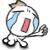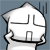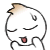#www.vustudents.ning.com

 www.bit.ly/vucodes + Link For Assignments, GDBs & Online Quizzes Solution www.bit.ly/papersvu + Link For Past Papers, Solved MCQs, Short Notes & More

 Assignment No. 02 Semester: Fall 2014 Compiler Construction CS606 Total Marks: 20   Due Date: 08/12/2014 Objective:   To learn and understand basic concepts of Parse Trees, Top Down Parsers, Predictive Parsing, First and Follow Sets in building a Lexical analyzer. Instructions: It should be clear that your assignment will not get any credit (zero marks will be awarded) if:   The assignment is submitted after due date. The submitted assignment does not open or file corrupt. The assignment is copied (from other student or copy from handouts or internet). Student name and ID are not mentioned in the assignment file. It is in some format other than .doc or .docx(MS Word Document). For any query about the assignment, contact at cs606@vu.edu.pk Question No 1:                                                                                                                       Marks 10 Consider the following grammar; you are required to write left most derivation of the string “aebb”.             Question No 2:                                                                                                                        Marks 10              Consider the following grammar for arithmetic expressions:   A à id X X àA Y |ε Y à- X | + X | / X | * X   Show the first set for the non-terminals X and Y in the grammar. BEST OF LUCK

+ http://bit.ly/vucodes (Link for Assignments, GDBs & Online Quizzes Solution)

+ http://bit.ly/papersvu (Link for Past Papers, Solved MCQs, Short Notes & More)

Views: 1775

### Replies to This Discussion

Question 2 ka First and follow set ka solution hai kisi k pasMay it could be helpful for 2nd question..

## first(x) for all grammar symbols x

Apply following rules:

1. If X is terminal, FIRST(X) = {X}.
2. If X → ε is a production, then add ε to FIRST(X).
3. If X is a non-terminal, and X → Y1 Y2 … Yk is a production, and ε is in all of FIRST(Y1), …, FIRST(Yk), then add ε to FIRST(X).
4. If X is a non-terminal, and X → Y1 Y2 … Yk is a production, then add a to FIRST(X) if for some i, a is in FIRST(Yi), and ε is in all of FIRST(Y1), …, FIRST(Yi-1).

Applying rules 1 and 2 is obvious. Applying rules 3 and 4 for FIRST(Y1 Y2 … Yk) can be done as follows:

Add all the non-ε symbols of FIRST(Y1) to FIRST(Y1 Y2 … Yk). If ε ∈ FIRST(Y1), add all the non-ε symbols of FIRST(Y2). If ε ∈ FIRST(Y1) and ε ∈ FIRST(Y2), add all the non-ε symbols of FIRST(Y3), and so on. Finally, add ε to FIRST(Y1 Y2 … Yk) if ε ∈ FIRST(Yi), for all 1 ≤ i ≤ k.

Example:

Consider the following grammar.

`E → E + T | T`
`T → T * F | F`
`F → (E) | id`

Grammar after removing left recursion:

`E → TX`
`X → +TX | ε`
`T → FY`
`Y → *FY | ε`
`F → (E) | id`

For the above grammar, following the above rules, the FIRST sets could be computed as follows:

FIRST(E) = FIRST(T) = FIRST(F) = {(, id}

FIRST(X) = {+, ε}

FIRST(Y) = {*, ε}

sorry,abhi kuch keh nahi sakti..thanks for sharing old file..

mujhay b kuch aesa hi lag rha hai..but...

can you explain 1st ques from old solution file?

if S ―> aS | Ab

then how it can be?

First (S) = {a, b, c, d , e}

sorry mujy b nahi pta kesy aya . ma to bus compare kr k ans bta raha hon .

Upload kr dia hai assignment . Ab Allah kher kry kia bnta haiassignment upload karo plzok.

can you explain 1st ques from old solution file?

if S ―> aS | Ab

then how it can be?

First (S) = {a, b, c, d , e}

مَلیکا ایمان appi.

S ―> aS | Ab

A ―> XYZ |ε

Yh islay hy  Q ky A ky andr XYZ hy Ju yh show kr rhy hain

a,b tu S ky apny hu Gy Hain aur c,d,e XYZ ky hu gy hain wo

isLy hoWy HaiN q ky S ky andr A nonterminal Show raha hy aur

A ky andr XYZ show hu rahy hndear..explain some logic ..k ye alphabetics kis logic k through or kyun aa rhay hein??

simple si bat hy Appi ju Terminal hain wo 1st sets bny gy

then iska First just {a,b} hona chahiaye na k  {a,b,c,d,e}...